### 一、4*4键盘矩阵

4×4 键盘矩阵共有 16 个按键，按照“线与”的交叉连接方式排成一个矩阵，即 16 个按键分别连接在 4 行 4 列导线的交叉点上。单片机系统按键如果采用矩阵连接方式，可以节省一半的 I/O 资源。

### 二、仿真

#### 1、选择元器件

这次实验要用的元器件有：AT89C51、按钮、两个共阳极数码管。两个共阳极数码管选择位置如下：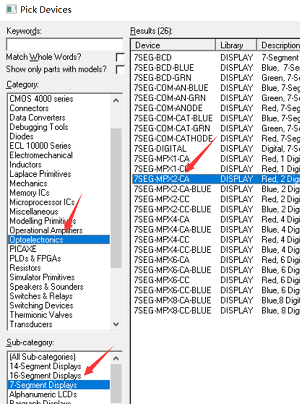#### 2、摆放模拟电路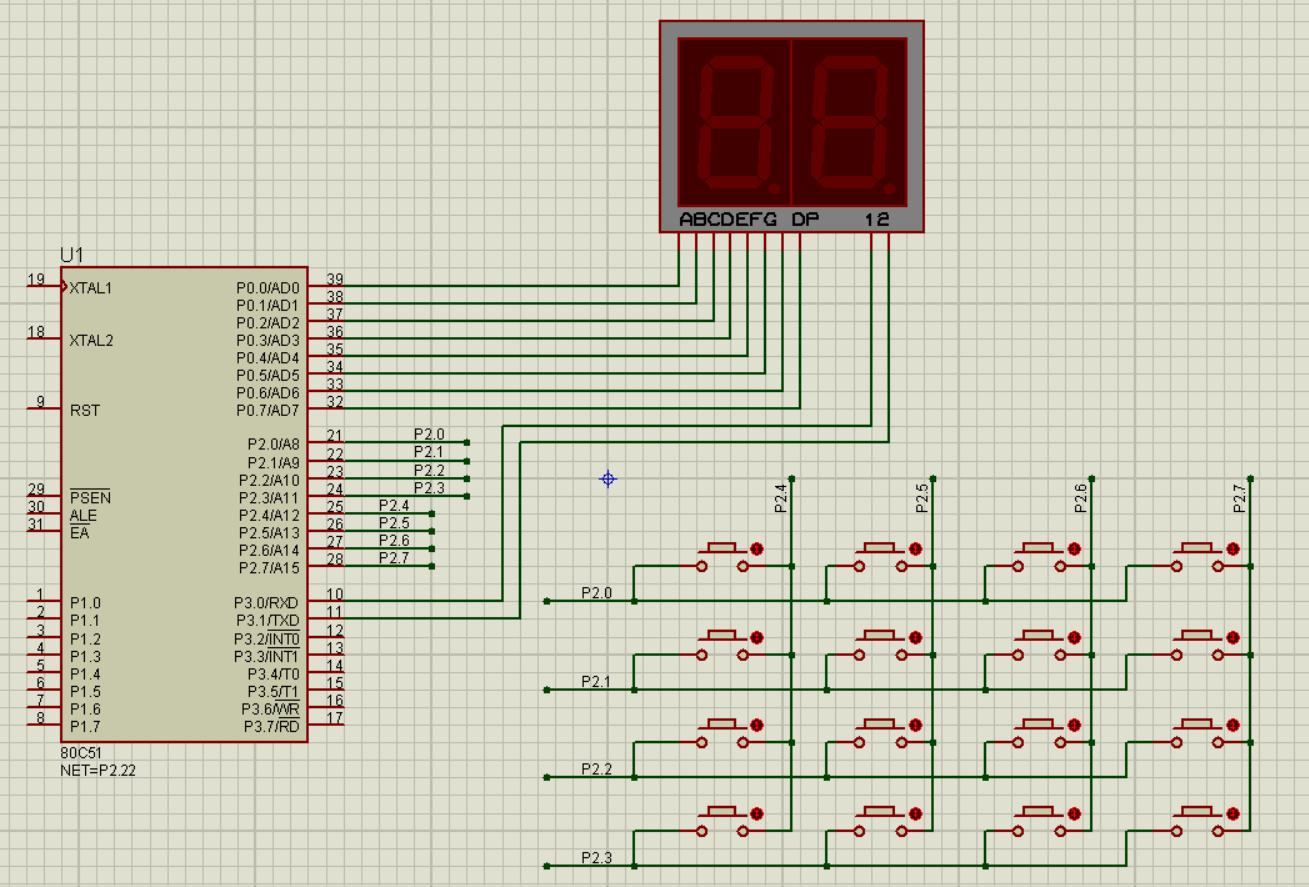##### 网络标号

上图中按键和 P2 接口之间没有直接用导线连接，而是采用具有电气特性的网络标号。在电路设计过程中，加注同一标号的导线，电路系统认为是连在一起的。在设计单片机系统或其他电子系统的电路时，由于元器件引脚较多，电路分成几个模块，元器件与元器件之间经常采用网络标号。

首先点击下图的按钮。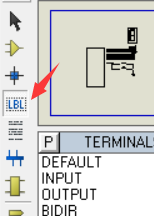然后按 a，弹出模态框，输出以下内容，点击 OK。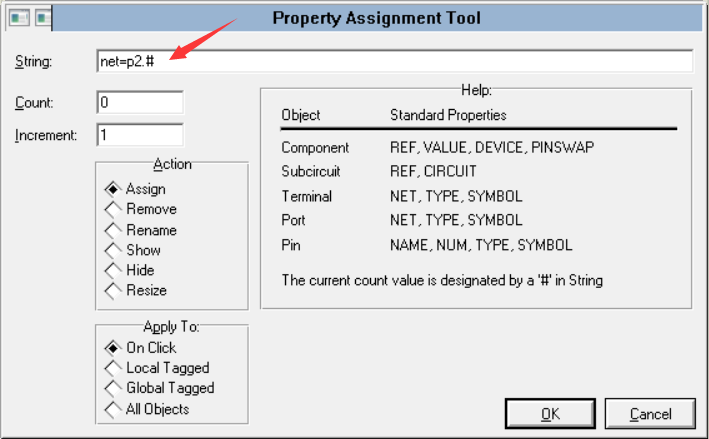依次点击要标号的导线即可。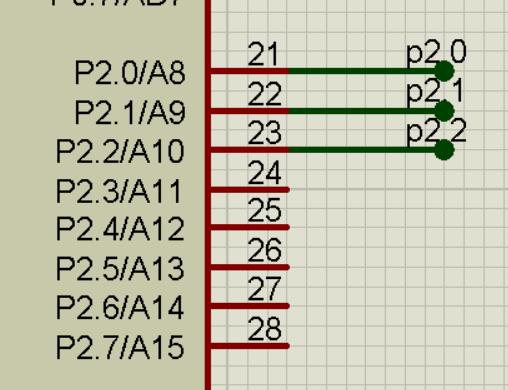### 三、程序

4*4 键盘矩阵的程序设计采用了 I/O 接口扫描与检测原理。按键处理过程首先让 P3 输出按键扫描数据，然后检测按键是否按下，经判断后返回与按键对应的键值。单片机的 P0 口用来控制数码管显示的数字；P2 口用来检测用户按下了哪个按键，从而进行相应的操作；P3.0 控制数码管显示的个位，P3.1 控制数码管显示的十位。

#### 1、动态显示原理

如果让数码管只显示个位，P3.0 输出高电平，P3.1 输出低电平，并且此时 P0 口输出个位要显示数字的二进制；十位同理。以此循环，十位数码管显示，然后回来再显示个位。这种利用 2 分时控制实现的多位数码管显示方式为动态显示。只要每个数码管显示的交替时间足够的快，由于人的视觉暂留特性，我们看到的结果是一个两位数。

#### 2、消隐

数码管在进行动态显示数字时，会出现显示错乱。这是数码管动态扫描显示方式造成的：当你在数码管上循环显示 1，2 时，因为人有视觉暂留，当显示完 1 后，再显示 2，如果间隔时间过短，那就会感觉 1、2 两个数字是叠加在一起显示的。如果你在两位数码管上显示，那就会两位同时显示出叠加字 —— 重影。
如何消隐：单片机要做的是，当第一位显示完 1 后，给出信号关闭数码管，然后再第二位显示 2，就不会出现重影了。

#### 3、代码实现

`````` 1#include<reg51.h>
2code unsigned char seven_seg[]={0xc0,0xf9,0xa4,0xb0,0x99,0x92,0x82,0xf8,0x80,0x90};
3unsigned char k=56;
4sbit P2_4=P2^4;
5sbit P2_5=P2^5;
6sbit P2_6=P2^6;
7sbit P2_7=P2^7;
8
9void delay(unsigned int x){while(x--);}
10void display(void)
11{
12	//消隐
13	P0=0xff;
14	//获取个位
15	P0=seven_seg[k%10];
16	//显示个位
17	P3=0x02;
18	delay(500);
19	P0=0xff;
20	//获取十位
21	P0=seven_seg[k/10];
22	//显示十位
23	P3=0x01;
24	delay(500);
25}
26void main(void)
27{
28	while(1)
29	{
30		display1();
31		//扫描每个端口，先将 P2.0口设为低电平，如果P2.4-6中有为低电平，则表示P2.0行有按钮被按下
32		P2=0xfe;
33 		while(P2_4==0)k=0;
34		while(P2_5==0)k=1;
35		while(P2_6==0)k=2;
36		while(P2_7==0)k=3;
37
38		P2=0xfd;
39 		while(P2_4==0)k=4;
40		while(P2_5==0)k=5;
41		while(P2_6==0)k=6;
42		while(P2_7==0)k=7;
43
44		P2=0xfb;
45 		while(P2_4==0)k=8;
46		while(P2_5==0)k=9;
47		while(P2_6==0)k=10;
48		while(P2_7==0)k=11;
49
50		P2=0xf7;
51 		while(P2_4==0)k=12;
52		while(P2_5==0)k=13;
53		while(P2_6==0)k=14;
54		while(P2_7==0)k=15;
55	}
56}
``````